## Main

Solitons are localized waves arising from nonlinear and dispersive interactions, and are central objects of nonlinear science. The well-known envelope solitons of the NLSE have been studied in many different systems including plasmas, optical fibres and cold atoms8,9,10. In addition to envelope solitons, the NLSE admits other classes of localized structure, and there has been significant interest in spatio-temporal breather solutions that undergo periodic energy exchange with a finite background11,12. However, despite extensive mathematical studies4,5, experiments have been limited to only a small number of discrete systems9,13. Indeed, to our knowledge no studies have explicitly characterized nonlinear breather localization in any system described by the continuous NLSE. As a result, predictions such as Peregrine’s that are central to nonlinear wave theory have remained untested.

In a sense, this is surprising because the theory of NLSE breather evolution also describes induced modulation instability, a process extensively studied in hydrodynamics and fibre optics14,15,16,17,18. Experiments in optics, however, have been strongly motivated by telecommunications goals to generate high-contrast pedestal-free pulses19,20,21,22, and the opportunity to characterize solitons on a finite background seems to have been overlooked. Indeed, even fundamental studies of Fermi–Pasta–Ulam recurrence in modulation instability have been carried out using initial conditions far from those that would excite Peregrine soliton features23. Here, we report experiments in optical fibre specifically designed to study breather evolution in a regime approaching the excitation of the Peregrine soliton. We demonstrate explicitly its spatio-temporal localization and, at the point of maximum temporal compression, use frequency-resolved optical gating (FROG) to explicitly measure the temporal soliton characteristics on a finite background. Our results are in very good agreement with numerical simulations and Peregrine’s analytic prediction.

Our experiments are designed using the breather formalism of ref. 2. With dimensionless field ψ(ξ,τ), the self-focusing NLSE is:Here ξ and τ are normalized distance and time, and induced modulation instability involves the evolution along ξ of a temporally modulated continuous wave towards a train of ultrashort compressed pulses followed by a return phase of broadening towards the initial state. Although the general evolution can be complex, it has recently been shown24 that the compression dynamics can be described for a wide range of initial conditions by the analytic Akhmediev breather2,4: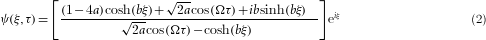Here Ω is the dimensionless modulation frequency, a=1/2(1−Ω2/4), where 0<a<1/2 determines the frequencies that experience gain and b=[8a(1−2a)]1/2 determines the instability growth. Dimensional transformations are given below, and dimensional forms of both equations (1) and (2) are given in the Methods section.

Figure 1 shows how the breather characteristics depend strongly on modulation frequency. As the modulation parameter a increases, the temporal separation between adjacent peaks increases at the same time as the compressed temporal width of each individual peak decreases. This leads to a greater temporal localization (defined below) as a approaches the limiting value of 1/2. The evolution at a=1/4 is associated with maximum modulation-instability gain, and is indeed the regime of previous experiments19,23. The limiting solution for a→1/2 derived by Peregrine has a particular fractional form that has led this class of solution to be described as a ‘rational soliton’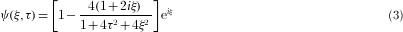The figure also plots the ideal Peregrine soliton to show the breather evolution approaching this solution as the overlap between adjacent peaks reduces with increasing a.Figure 1: Plotted Akhmediev breather solutions using equation (2) for modulation parameter a=0.25, a=0.45 and a=0.48, as well as the ideal Peregrine soliton of equation (3), the limiting case of the Akhmediev breather as a→1/2.

Figure 2a shows in more detail the dependence on modulation parameter of the breather profile at the point of maximum temporal compression ξ=0. The pseudocolour plot ofhighlights the increased peak localization as a→1/2, with the right panels illustrating how the intensity of the half-period breather peak (shaded region delimited by arrows) approaches the Peregrine soliton (solid line) for a>0.4. These results are important in showing that this parameter regime yields characteristic Peregrine soliton features in the temporal envelope even though the ideal solution exists only asymptotically in the limit of zero modulation-instability gain (b→0 as a→1/2).Figure 2: Evolution towards Peregrine soliton characteristics with increasing modulation parameter for temporal profile characteristics and localization behaviour.

The emergence of Peregrine soliton characteristics with increased temporal localization is also associated with increasing spatial localization. This is because the modulation-instability recurrence period also increases asymptotically as a→1/2. To discuss this quantitatively, we introduce localization measures in terms of ratios of the temporal and spatial periods relative to the individual temporal and spatial peak half-widths. These can be readily calculated or determined from numerical simulations of the NLSE as a function of modulation parameter (see the Methods section). The solid lines in Fig. 2b plot (i) temporal localization τper/δ τo, (ii) spatial localization ξper/δ ξo and (iii) their product (τper/δ τo)(ξper/δ ξo), which defines spatio-temporal localization. The regime of rapidly increasing ‘strong localization’ for a>0.4 is where the right panels in Fig. 2a show Peregrine soliton characteristics in the temporal envelope. As discussed below, this regime is accessible in our experiments. This analysis is thus highly significant because it shows how Peregrine soliton characteristics can appear experimentally, even though the ideal mathematical asymptotic limit can never be reached in practice.

Figure 3 shows our experimental set-up (see the Methods section). An input fieldis injected into fibre with group velocity dispersion β2(s2 m−1) and nonlinearity γ(W−1 m−1). The input power is P0, and αmod and ωmod are modulation strength and frequency. The dimensional field A(z,T)(W1/2) is A=P01/2ψ. Defining a characteristic length LNL=(γ P0)−1 and timescale T0=(|β2|LNL)1/2, dimensional distance z (m) and time T (s) are related to the normalized parameters by z=ξ LNL and T=τ T0. The frequency ωmod is related to the modulation parameter a by 2a=[1−(ωmod/ωc)2] with ω2c=4γ P0/|β2|. Modulation-instability gain is seen for modulation parameters 0<a<1/2, which corresponds to ωc>ωmod>0. With these definitions, evolution towards the Peregrine soliton as a→1/2 corresponds to the limit where ωmod→0, accessible in practice by beating two narrow-linewidth lasers to create an initial low-frequency-modulated wave.

We used an input field obtained from a pump laser at λp=1,554.53 nm mixed with a closely spaced tunable signal at λs, with αmod=0.225 (see the Methods section for other parameters). Experiments first studied two-dimensional localization dynamics as a function of modulation parameter a. Although we use a fibre of fixed length, the spatial dynamics in ξ were readily measured by varying pump power (recall ξ=z γ P0). By changing the pump–signal detuning to vary a while studying dynamical evolution by varying P0, the temporal and spatial localization parameters defined above were determined from the ξ and τ dependence of the measured autocorrelation function g(ξ,τ)=〈|ψ(ξ,t)|2 |ψ(ξ,tτ)|2〉. Figure 2b shows that the measured localization parameters are in very good agreement with predictions. This verifies that we can enter experimentally the divergent regime where Peregrine soliton characteristics are observed with the values of a>0.4 accessible with our set-up.

Figure 4 shows detailed measurements of dynamics and the compressed temporal profile for strong localization with a=0.42. Figure 4a shows simulations plotting the expected evolution of |ψ(ξ,τ)|2 over the range of ξ varied in our experiments (see the Methods section). Figure 4b,c shows the simulated autocorrelation function and spectrum. These results are compared to experiment (with no free parameters), exhibiting very good agreement. The autocorrelation results in Fig. 4b (i) are particularly clear in showing the two-dimensional localization at the distance of maximum compression when ξ=2.5. To illustrate the growth–decay intensity dynamics explicitly, Fig. 4b (ii) shows an increase in autocorrelation peak signal with ξ. Here, experiments (points) are compared with simulations (line).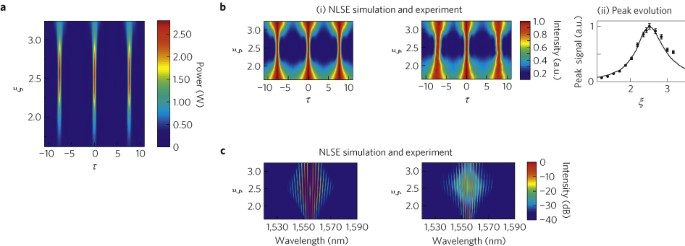Figure 4: The variation in temporal and spectral characteristics with normalized propagation distance to confirm expected dynamical evolution.

Detailed temporal measurements using FROG were carried out at ξ=2.5 where Peregrine soliton characteristics are expected at maximum compression. Figure 5a,b shows the measured and retrieved FROG traces. The retrieved intensity and phase (see the Methods section) are shown as the blue line with markers in Fig. 5d. The peak power of the retrieved profile is calculated from the measured output power with no free parameters. Experimental results are compared to numerical simulations (red line), and we see excellent agreement. Figure 5c shows the FROG trace of the simulated field. The grey line plots the analytic Peregrine soliton from equation (3), with maximum peak power from theory of 9P0=2.7 W. On the right axis, the intensity for all curves is normalized to that of the dimensionless.Figure 5: Experimental results showing the measured temporal characteristics of the maximally compressed pulse at ξ=2.5, and comparison with the predicted Peregrine soliton.

These measurements confirm the expected temporal features of the Peregrine soliton—a temporally localized peak (400 fs duration) surrounded by a non-zero background. The FROG measurements also confirm the different signs of the peak and background amplitudes through the measured relative π phase difference. The measured phase profile (blue line and markers) in the vicinity of the intensity null (indicated by the arrow) is shown in Fig. 5d and compared to that expected for the Peregrine soliton (grey line). Finally, in Fig. 5e we plot the measured spectral intensities (blue) compared to simulation (red markers), and the analytic spectrumfor the ideal Peregrine soliton (grey). Note that this spectrum is calculated for the time-varying envelope component so that the delta-function component at the pump is not shown, but the analytic spectrum reproduces very well the decay of the measured sideband amplitudes.

These experiments represent the first amplitude and phase measurements of a nonlinear breather structure in any continuous NLSE soliton-supporting system. The results show the existence of a strongly localized temporal peak on a non-zero background, and confirm Peregrine’s theoretical predictions of a rational soliton envelope. Our results highlight how experiments in optics using readily available components and advanced pulse metrology can be used to conveniently test more general theories of nonlinear waves 25. We anticipate applications in establishing links between optical and hydrodynamic extreme events26 and in studying modulation-instability dynamics in other systems27. In showing that Peregrine soliton characteristic are excited even for non-ideal perturbation frequencies, our results may impact on the search for oceanic rogue-wave forecasting signatures in meteorological data.

## Methods

For completeness, we first give dimensional forms of the NLSE and breather solutions equations (1) and (2) respectively. The dimensional NLSE is given by: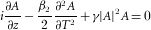with β2<0 for the self-focusing form (that is, fibre anomalous dispersion). The breather evolution in z and T is given in dimensional form as: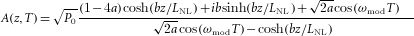The numerical results in Figs 1 and 2 were obtained directly from equation (2). In numerical simulations solving the NLSE equation (1), we used a standard split-step scheme28.

The localization properties shown in Fig. 2 are defined in terms of normalized variables as follows. A measure of time-domain localization can be derived analytically from the form of profile of the breather solution at the point of maximum temporal compression:. We define the profile temporal width δ τ0 as the position when the intensity is zero-valued adjacent to the peak. Zeros appear in the profile for a>1/8, and the ratio between the period τper=2π/Ω and the temporal width can then be expressed analytically as:. This expression is that used to plot the solid line in Fig. 2b (i). Under non-ideal initial conditions, multiple spatial recurrence periods of breather evolution are observed, and longitudinal localization is not amenable to straightforward analysis. We therefore used numerical simulations of the NLSE plotting peak intensity evolution with ξ. This allows us to determine the spatial period ξper and the half-width δ ξo of the intensity evolution with ξ to yield the ratio ξper/δ ξo. The solid line in Fig. 2b (ii) shows a smooth polynomial fit to simulations that we carried out for varying a. At the parameters used in our experiments for the detailed temporal analysis (a=0.42), the measured spatial localization factor exceeded 10.

In our experiments, the initial signals (pump and seed) were generated from two telecommunications-grade external-cavity lasers (ECL-OSICS model 1560-PM) with intrinsic linewidths <200 kHz. The fibre used was 900 m of highly nonlinear fibre (OFS Specialty Fiber) with β2=−8.85×10−28 s2 m−1 and γ=0.01 W−1 m−1 at λp. The fibre was dispersion-flattened to have low third-order dispersion β3=1.331×10−41 s3 m−1 around 1,550 nm. Fibre loss was 1 dB km−1. A phase modulator was used to broaden the narrow intrinsic external-cavity-laser linewidths to 100 MHz so as to suppress Brillouin scattering in the fibre at the power levels used in our experiments28. Both the pump and the seed were then amplified to the power levels used in the experiments by means of an erbium-doped fibre amplifier (EDFA-IPG model EAD-1-C-PM). Note that the injection set-up was all-polarization maintaining to maximize the modulation-instability process occurring in the optical fibre. A low-noise amplifier was used so as to clearly favour the induced breather dynamics over spontaneous broadband modulation instability. Indeed, the limiting factor in reducing the modulation frequency between the pump and the signal so as to approach the ideal case of a→1/2 is the decreasing gain for the stimulated process relative to the spontaneous growth of sideband content, which occurs over a continuous range from the pump to the maximum frequency ωc. In our experiments this limited the maximum attainable value of a=0.42.

For the experimental results in Fig. 4, we varied P0 from 0.2 to 0.4 W while varying ωmod over 196.7–278.2 GHz (by tuning λs from 1,556.12–1,556.77 nm) to maintain constant a=0.42. Note that the spectral measurements in Fig. 4 are plotted against wavelength to show the change in modulation frequency that ensures that a is held constant. The point of maximum temporal compression at ξ=2.5 corresponded to P0=0.30 W and ωmod=241 GHz. For the results in Fig. 5, the FROG technique used a second-harmonic generation implementation, and retrieval of the intensity and phase of the underlying field from the measured FROG trace was carried out using a generalized projections algorithm, adapted for finite background fields and/or periodic pulse trains 29. We measured five periods of the FROG trace although for clarity only the central period is shown in Fig. 5. The non-collinear autocorrelation measurements were carried out using an independent second-harmonic generation autocorrelator providing an important verification of measurement fidelity. Both autocorrelation and FROG measurements are self-referencing and thus insensitive to relative source phase variation. Retrieval was carried out using a 256×256 grid and the FROG error for the result in Fig. 5d was G=0.005, a typical error for a periodic FROG trace29. Optical spectra were measured using a 0.02-nm-resolution bandwidth optical spectrum analyser (Yokogawa-AQ6370). In practice, optimization of the injected power to obtain maximum compression for the available fibre length of 900 m was used by continuous monitoring of spectral and autocorrelation measurements for various input powers around 0.3 W.

The NLSE simulations shown in Fig. 4 for our experimental conditions considered an input corresponding to a weakly modulated continuous-wave field with modulation frequency and depth corresponding to experiment. A low level of bandwidth-limited noise at −50 dB modelled the effect of amplified spontaneous emission in the EDFA and a phenomenological one photon per mode background was also included to model quantum noise30. The effect of fibre loss was included through a correction to the propagation distance (by using the effective length defined as Leff=[1−exp(−α L)]/α, where α accounts for fibre losses28) when plotting the experimental results. This approach is satisfactory in allowing the simulations to be used to confirm experimental observation of temporal compression and localization in the breather evolution. On the other hand, when comparing the retrieved intensity and phase of the Peregrine soliton, the simulations in Fig. 5 used generalized NLSE simulations including fibre third-order dispersion and spontaneous Raman scattering30. However, we found that the generalized NLSE simulations yield essentially the same temporal and spectral characteristics as a NLSE model at the power levels considered.